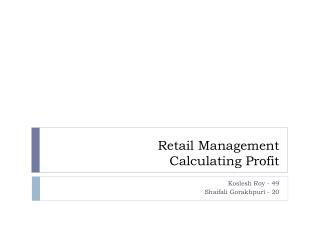DownloadDownload PresentationRetail Management Calculating Profit

# Retail Management Calculating Profit

Download Presentation## Retail Management Calculating Profit

- - - - - - - - - - - - - - - - - - - - - - - - - - - E N D - - - - - - - - - - - - - - - - - - - - - - - - - - -
##### Presentation Transcript

1. Retail ManagementCalculating Profit Koslesh Roy - 49 Shaifali Gorakhpuri - 20

2. Contents • Markup • Margin • Stock turn • Stock Productivity • GM ROI

3. Introduction • Half the art of selling, is the art of pricing • What price is fair for this customer? • What price will the market bear ? • Consider supplier discounts, list prices, VAT, acceptable margins • Decide a profitable Price • Terms to calculate Profit • Markup • Margin

4. Markup- Margin • Margin: • percentage of the final selling price that is profit. • Markup: • percentage of the cost price added on to get the selling price • Gross Profits • Overheads (like fixed costs, corporation tax, wages, etc) • Minimum acceptable margins.

5. Formula • MARKUP = (SP -CP) -------------- X 100 CP • MARGIN = (SP-CP) ----------- X 100 SP Markup belongs to cost and margin belongs to sales

6. Example: HUL offers Fixed Mark up of 6.66% on Tea; what price should you sell? • MRP of BROOKE BOND, RED LABEL is Rs 181/- • Markup = (SP – CP) / CP • 0.0666 x CP = SP – CP • 1.06666 CP = SP • Thus, CP = SP / 1.0666 • CP = 181/1.0666 = 169.7 RS • Margin = (181-169.7 ) / 181 = 6.24% • Margin = 5%; SP = ?? • Margin = (SP-CP) / SP • 0.05 SP = SP-CP • CP = (1- 0.05) SP • SP = CP / 0.95 = Rs179/- Sell BBRL Tea for Rs 179/- ; Gross Margin = 5%

7. Margin vs Markup Chart • 15% Markup = 13.0% Gross Profit • 20% Markup = 16.7% Gross Profit • 25% Markup = 20.0% Gross Profit • 30% Markup = 23.0% Gross Profit • 33.3% Markup = 25.0% Gross Profit • 40% Markup = 28.6% Gross Profit • 43% Markup = 30.0% Gross Profit • 50% Markup = 33.0% Gross Profit • 75% Markup = 42.9% Gross Profit • 100% Markup = 50.0% Gross Profit % Markup is always greater than % Margin

8. Markup & Margins vary from product to product and for different SKU to SKU • To calculate markups for non MRP Products markups are added to the sum of total costs (pdt + transportation + taxes etc)

9. STOCK TURN • Means rotation of STOCK in a year. • FORMULA = SALES / Avg Stock • Eg. Sale = Rs12,00,000/- • Opening Stock = Rs.50,000/- • Average Stock = Rs.50,000/- • Stock Turn = 24 Times • Stock Turn is always expressed in No. of Times.

10. WHAT IS THE IMORTANCE OF STOCK TURN RATE? • The Stock Turn Rate ratio measures the effectiveness of inventory planning control. • A Stock Turn Rate that is too low indicates poor planning and lack of control. • Stock Turn Rate can also be used to calculate the proper beginning-of-month inventory level for each classification on the Open-To-Buy.

11. STOCK PRODUCTIVITY • How much Gross Profit is generated by average stock. • Gross Profit in retailing is generated by cross force of MARGIN and STOCK TURN. • This is called ROSE – Return on Stock Employed. • Formula – 1) MARGIN X STOCK TURN 2) (GP X 100) / AVG.STOCK

12. GMROI • FORMULA = (GP X 100) / Paidup Stock • Gross Margin Return on Investment (GMROI) is a ratio in microeconomics that describes a seller's income on every dollar spent on inventory. • Rs100,000 annual profit) / (Rs25,000 average inventory cost) = GMROI of 4.0

13. THANK YOU